### Home > CALC > Chapter 5 > Lesson 5.2.1 > Problem5-54

5-54.
1. There is an ant moving along the edge of your table. Its position from the center of one edge of the table is given by the equation x(t) = (t − 1)(t − 4)4. When x(t) is increasing, the ant is moving forward. Homework Help ✎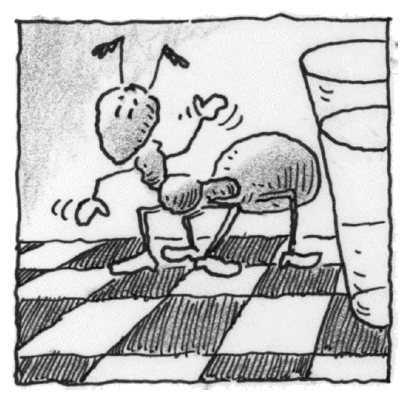1. Find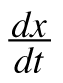.

2. If x(t) represents how many millimeters the ant is from the center of the table's edge after t seconds, what are the units for? What information does this derivative give about the ant?

3. What are the units for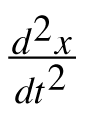? What information does this second derivative give about the ant?

4. How often does the ant stop and change direction? At what time(s)? Be sure to find and confirm your answer using both calculus techniques and a graph.

5. At time t = 3, is the ant moving forwards or backwards? Justify your answer using calculus.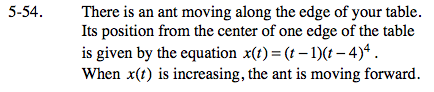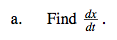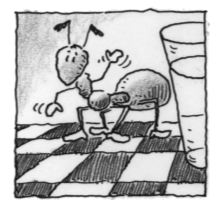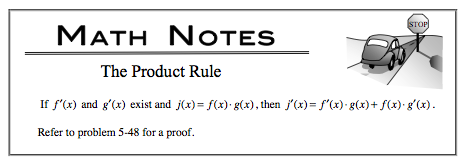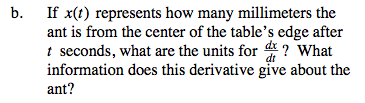$\text{Recall that }\frac{dx}{dt} \text{ means }\lim_{\Delta t\rightarrow 0}\frac{\Delta x}{\Delta t}.$

What does x and t stand for?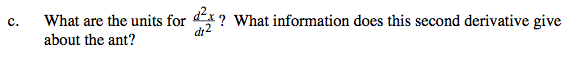Refer to the hint in part (b).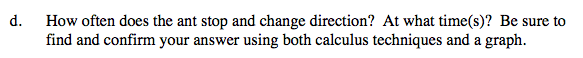Analytic method: The ant will change directions when x'(t) changes sign.
Graphical method: The ant will change directions when the derivative graph CROSSES the x-axis.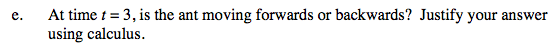When velocity is positive, the ant is moving forward. When velocity is negative, the ant is moving backwards.